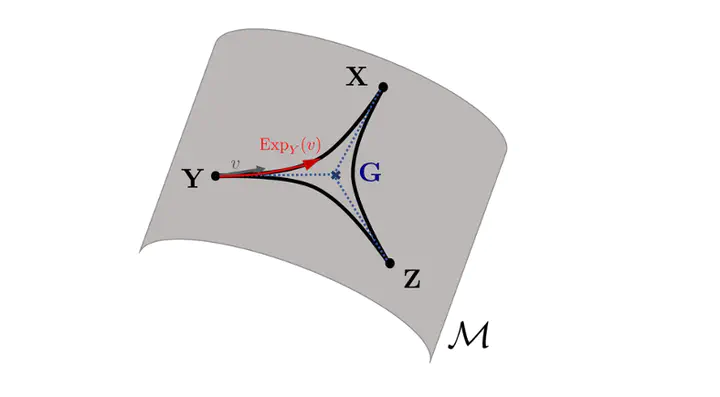# Optimization on ManifoldsMany applications involve non-Euclidean data, such as graphs, strings, or matrices. Exploiting such geometric structure in optimization can deliver algorithms that are computationally superior to standard nonlinear programming approaches. This observation has resulted in an increasing interest in Riemannian methods in the optimization and machine learning community.

This projects investigates the problem of optimizing a function on a (Riemannian) manifold subject to convex constraints. Several classical problems that arise in Machine Learning applications can be phrased as constrained optimization on manifolds. This includes matrix-valued tasks, such as barycenter problems, as well as the computation of Brascamp-Lieb constants. The latter is of central importance in many areas of mathematics and computer science through connections to maximum likelihood estimators in Gaussian models, Tyler’s M-estimator of scatter matrices and Operator Scaling.

We introduce Riemannian Frank-Wolfe methods, a class of first-order methods for solving constrained optimization problems on manifolds and present a global, non-asymptotic convergence analysis. We further analyze a class of CCCP-style algorithms for Riemannian “difference of convex” functions and explore connections to constrained optimization.

Code: GitHub